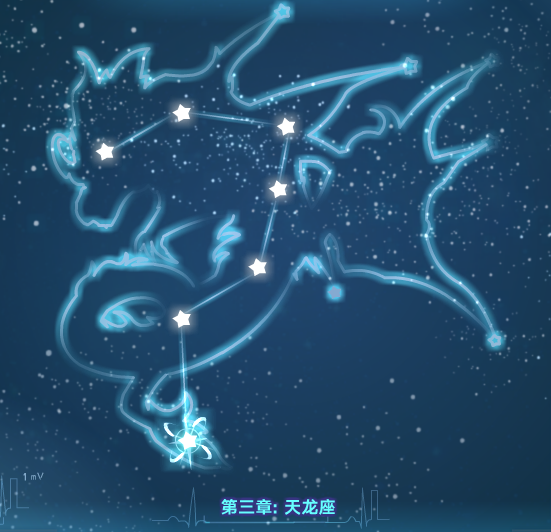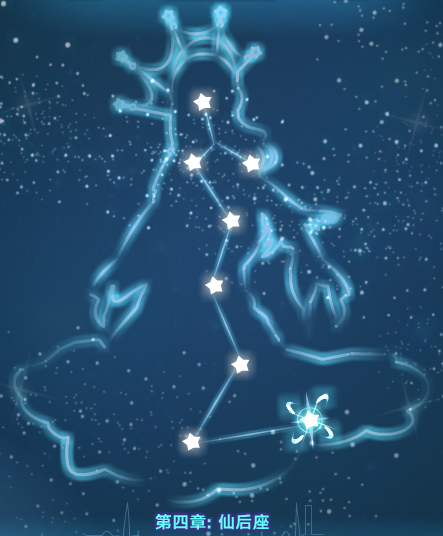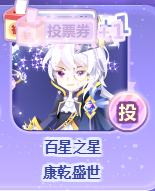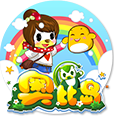奥比岛
攻略心得
热门奥剧
倾世狐缘
|
豪门千金：对战寒门之女
|
深海不知鱼有毒
|
我的五年级生活
|
今天长安还好吗
|
何以倾心 温暖入侵
|
行事轨迹
人气服饰
红宝石10周年甜心
|
最有价值的服装
|
全岛最耀眼套装
|
最值钱淑女装
|
公认最好看的套装
|
最新设计师作品
|
史上最帅的套装
免费福利
奥比岛微信每月福利
|
奥比岛金币怎么刷
|
免费得晶钻
|
每周装扮赛圈币
你现在的位置: 奥比岛 > 攻略心得 >星际旅行 神秘混沌第三、四章攻略

# 星际旅行 神秘混沌第三、四章攻略

作者：投稿用户   来源：百田网   时间：2020-06-15 10:22
这里星晨，奥比名：百星之星，这周给大家带来星际旅行 神秘混沌第三、四章攻略第三章：天龙星系第一关：土+水=能量； 能量+风=暴风； 水+石头=沙子； 沙子+暴风

这里星晨，奥比名：百星之星，这周给大家带来星际旅行 神秘混沌第三、四章攻略第三章：天龙星系

第一关：

土+水=能量；
能量+风=暴风；
水+石头=沙子；
沙子+暴风=沙尘暴。

第二关：

水+土=沼泽；
沼泽+沙子=黏土；
贝壳+石头=石灰石；
石灰石+黏土=水泥；
水泥+水=混凝土。

第三关：

水+石头=沙子；
沙子+火=玻璃；
玻璃+砂纸=放大镜；
石头+火=金属；
放大镜+金属=望远镜。

第四关：

沙子+生命=种子；
种子+能量=咖啡豆；
水+火=酒精；
酒精+水=伏特加；
伏特加+咖啡豆=鸡尾酒。

第五关：

能量+沼泽=生命；
生命+沼泽=细菌；
细菌+水=浮游生物；
浮游生物+土=蠕虫；
蠕虫+风=蝴蝶。

第六关：

火+风=能量；
能量+火=等离子；
等离子+太空=太阳；
水+水=大海；
太阳+大海=粗盐。

第七关：

水+风=蒸汽；
蒸汽+金属=锅炉；
树+火=煤炭；
锅炉+煤炭=蒸汽引擎。第四章：仙后星系

第一关：

家畜+草=化肥；

贝壳+石头=石灰石；

石灰石+化肥=硝石

原始生物+沼泽=硫磺；

硫磺+硝石=黑火药；

黑火药+武器=枪械。

第二关：

水+沙子=黏土；
黏土+太阳=砖头；
砖头+砖头=墙壁；
树+斧头=木屋；
木屋+墙壁=棚屋。

第三关：

木头+工具=轮子；
轮子+木头=拖车；
拖车+蒸汽引擎=机车；
水+煤炭=石油；
石油+机车=汽车。

第四关：

石头+火=金属 ；
金属+人类=工具；
人类+家畜=毛；
工具+毛=纺织物；
纺织物+胶水=创可贴。

第五关：

土+水=沼泽；
能量+沼泽=生命；
生命+沼泽=细菌；
人类+奶牛=牛奶；
牛奶+细菌=奶酪。

第六关：

蛋+能量=动物；
能量+沼泽=生命；
生命+动物=人类；
人类+文字=知识；
知识+罪恶=测谎仪。

第七关：

家畜+草=化肥；

石头+贝壳=石灰石；

化肥+石灰石=硝石；

细菌+沼泽=硫磺；

硝石+硫磺=黑火药；

黑火药+火=炸药。

第八关：

火山+风=岩石；
岩石+岩石=花火；
人类+树=树叶；
花火+树叶=篝火；
篝火+树叶=黑烟。最后再给自己拉拉票，星际大赛求支持。

本次攻略就到这里，下次再见

302400

### 看完这篇文章你有啥感想？已经有人表态

相关文章推荐
最新文章奥比岛官网

圈圈1190199个话题

简介: 奥比岛, 在一望无际的大洋上，有一个美丽神秘的奥比岛，小奥比们居住在里面，每天都发生一些好玩的故事。

仿冒网站的重要提示 奥比岛小说第二部发布预告 奖券商城玩法大揭秘